Physics
Fox

### Summary

• If an object's net force = 0, we say its forces are balanced.

• Balanced forces obey the balanced force law:
When an object's net force = 0, it continues to move at the same speed (and in the same direction).This car's speed stays the same because the net force = 0 (force forwards = force backwards)

• If an object's net force ≠ 0, we say its forces are unbalanced.

• Unbalanced forces obey the unbalanced force law:
F
$$\leftarrow$$
$$\rightarrow$$
F
When an object's net force ≠ 0, its speed increases (in the direction of the net force).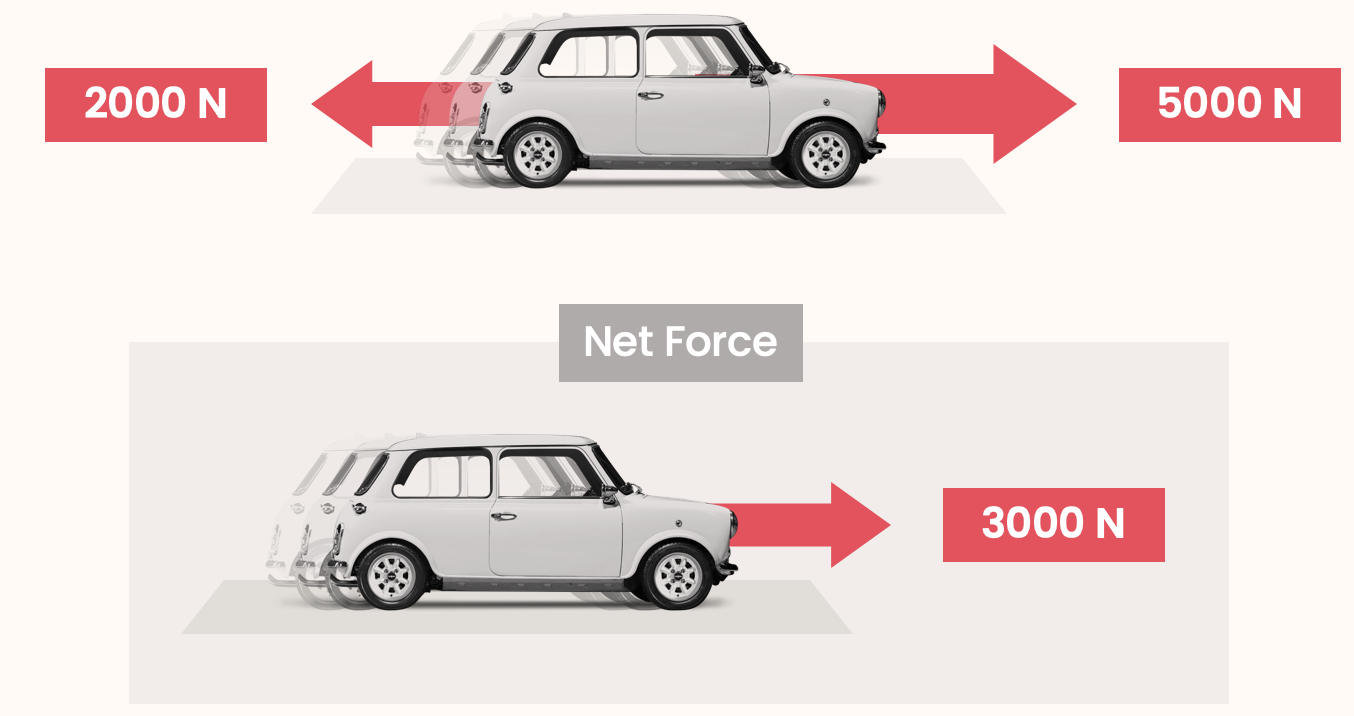This car's speed increases (i.e. it accelerates) because the force forwards > force backwards (so net force ≠ 0)

I feel perpetual
I'll feel perpetual

Now we know about speed, force, and net force, we can finally understand the balanced and unbalanced force laws.

What does it mean for forces to be balanced or unbalanced? This depends on the net force: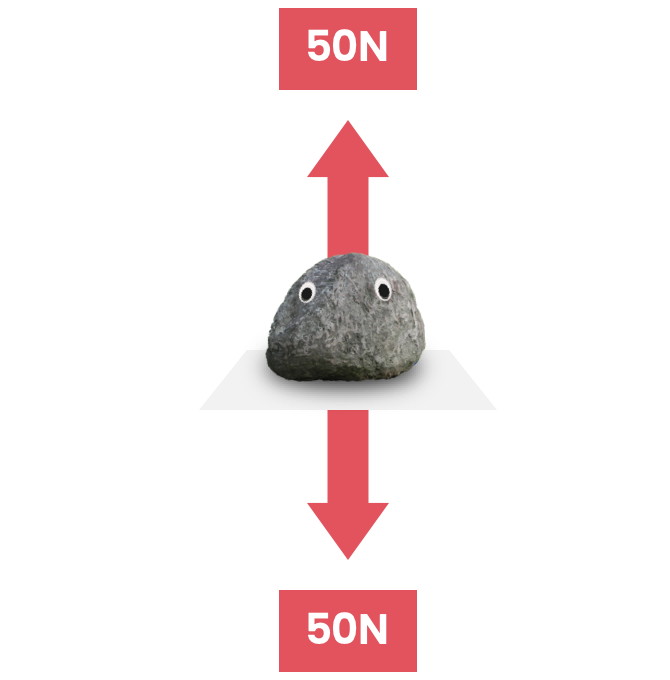If an object's net force = 0, we say its forces are balanced.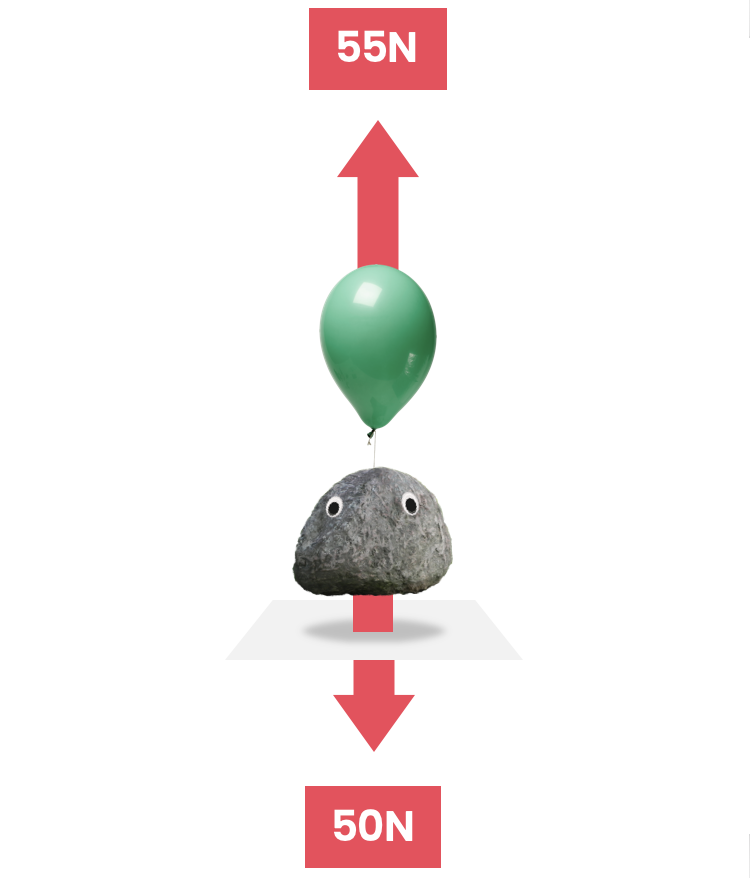If an object's net force ≠ 0, we say its forces are unbalanced.

Let's recall the balanced force law from the beginning of this chapter: When an object's net force = 0, it continues to move at the same speed (and in the same direction). In other words, when an object's forces are balanced, it's speed won't change.

This law is best understood with some examples: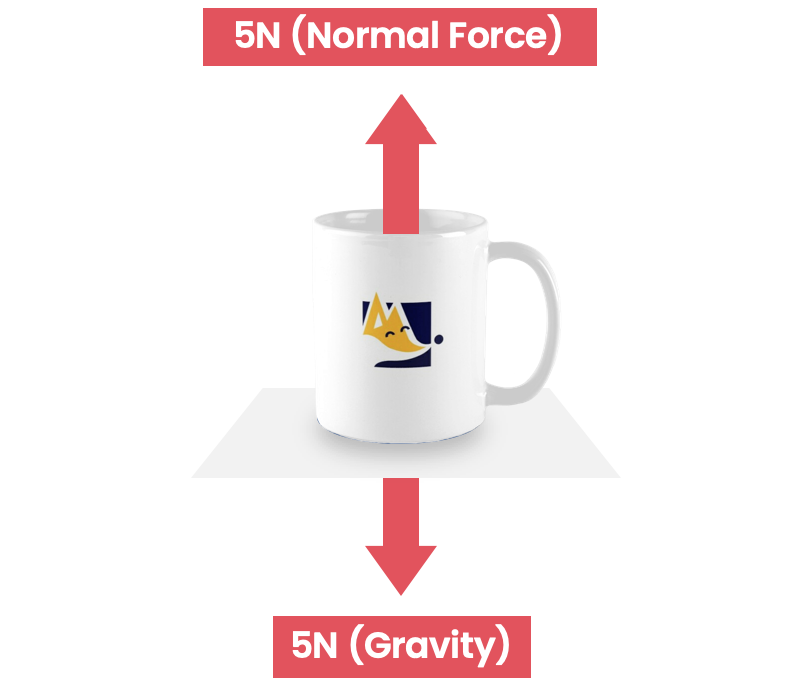Speed = 0. Gravity down = normal force up, therefore the net force = 0 (i.e. the forces are balanced). Because of the balanced force law, the speed continues to be 0!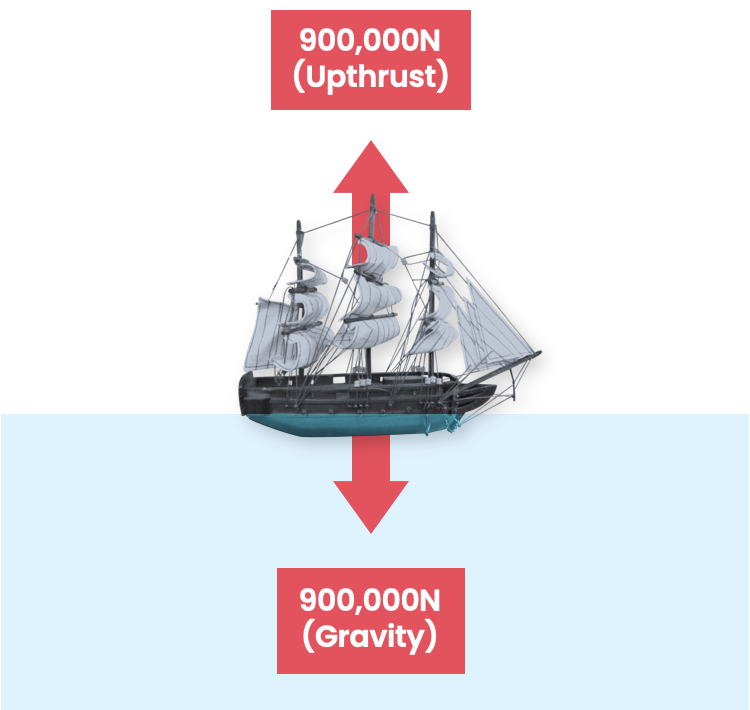Speed = 0. Gravity down = upthrust up, therefore the net force = 0 (i.e. the forces are balanced). Because of the balanced force law, the speed continues to be 0!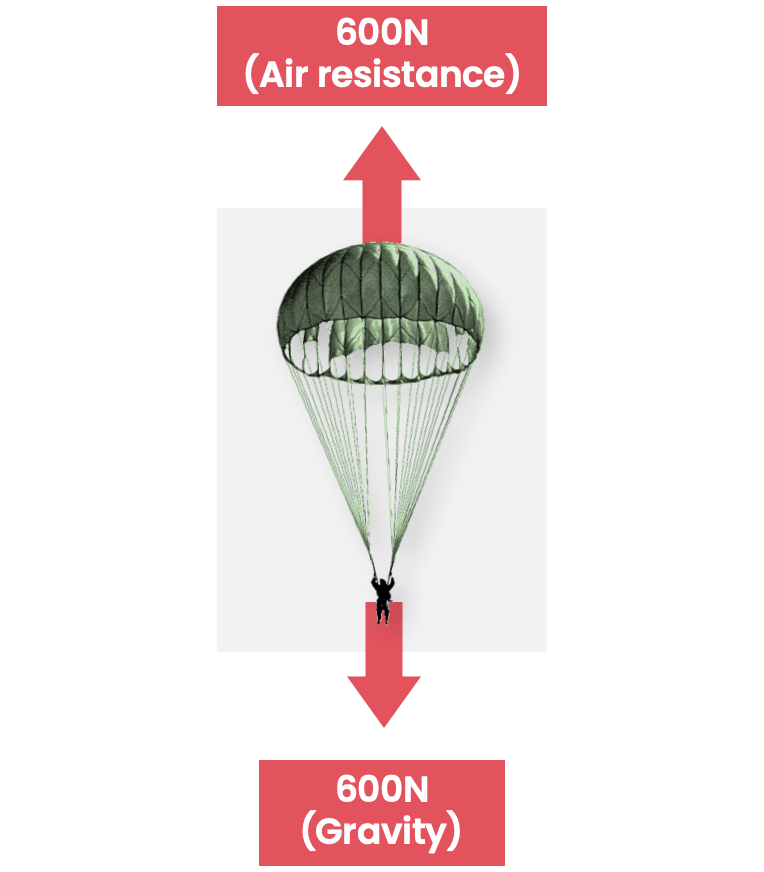Moving downwards at some speed (say 5 m/s). Gravity down = air resistance up, therefore the net force = 0 (i.e. the forces are balanced). Balanced force law means that the speed will not change, so the parachute continues moving downwards at 5 m/s.

Let's also recall the unbalanced force law: When an object's net force ≠ 0, its speed increases (in the direction of the net force).

This sounds complicated, but it's actually something you're familiar with. All it is really saying is that when something is pushed, it moves more (in the direction of the push). This is easy to notice when pushing a shopping trolley: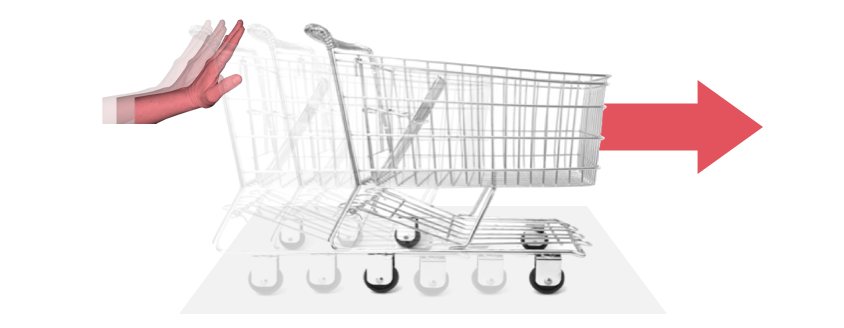Once again, this law is best understood with some examples: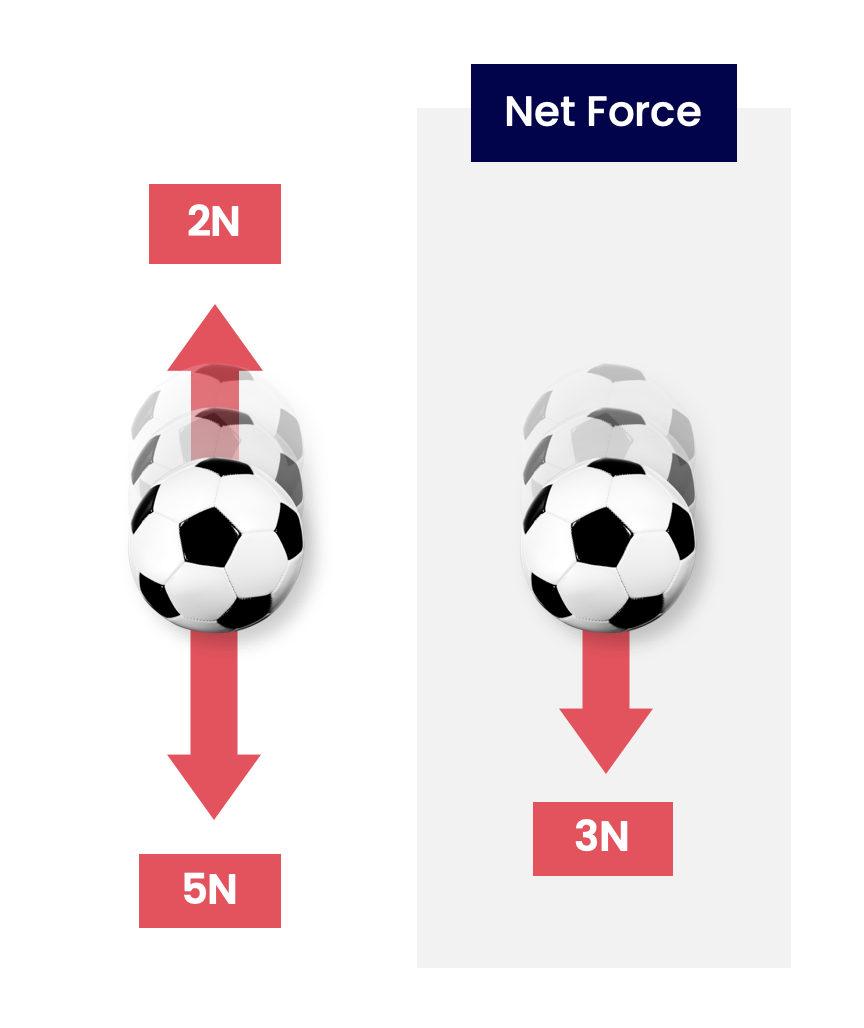The ball is moving downwards at some speed. Unlike the parachute above, the gravitational force down is larger than air resistance up. Therefore the net force ≠ 0 (i.e. the forces are unbalanced). Because of the unbalanced force law, the ball's (downward) speed increases. This is what you'd expect!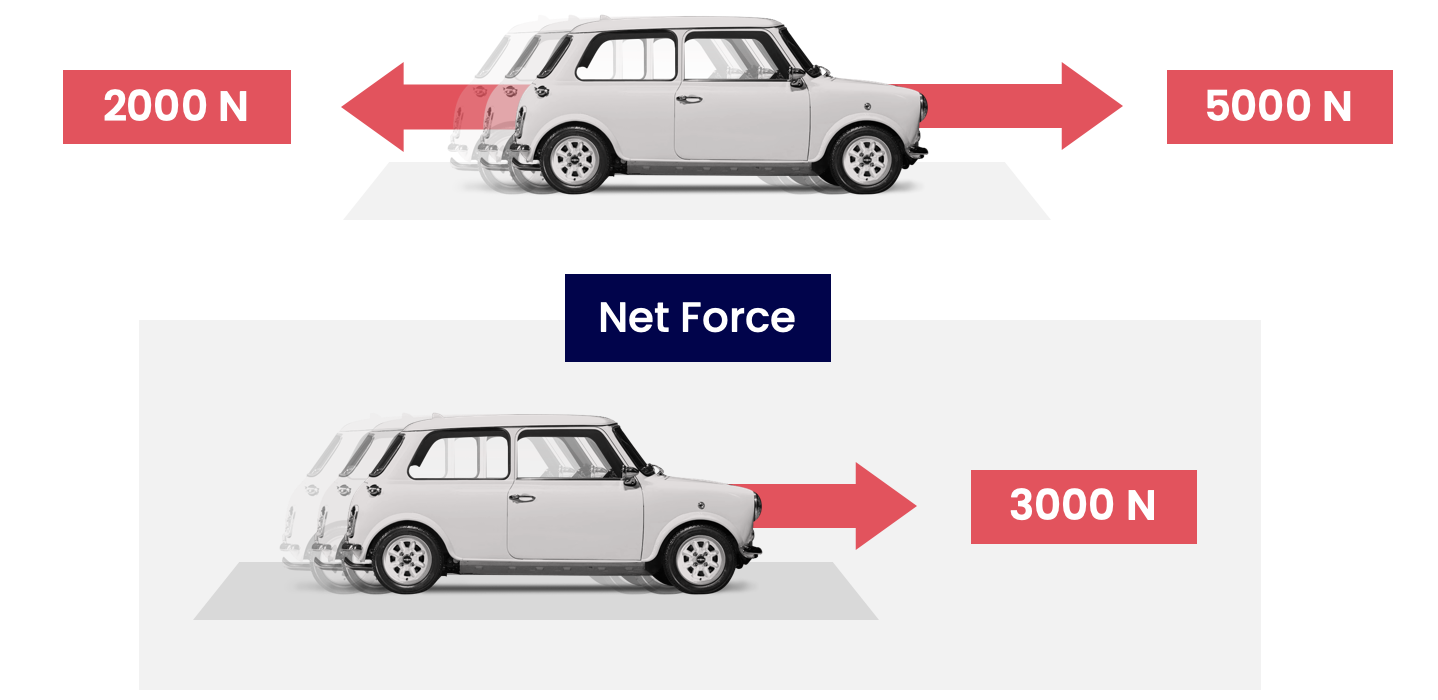The car is moving to the right at some speed. The thrust forwards is larger than the air resistance backwards. Therefore the net force ≠ 0 (i.e. the forces are unbalanced). Because of the unbalanced force law, the car's (forward) speed increases.

An increase in speed is also known as acceleration. Racecars have a large acceleration, so they can go from a low to high speed in a short amount of time.

Did you know that the word ‘racecar’ is the same forwards as it is backwards? Madness.Here's something that might seem obvious, but it also an important law of physics:

When a heavy thing is pushed, it moves less than a lighter thing would.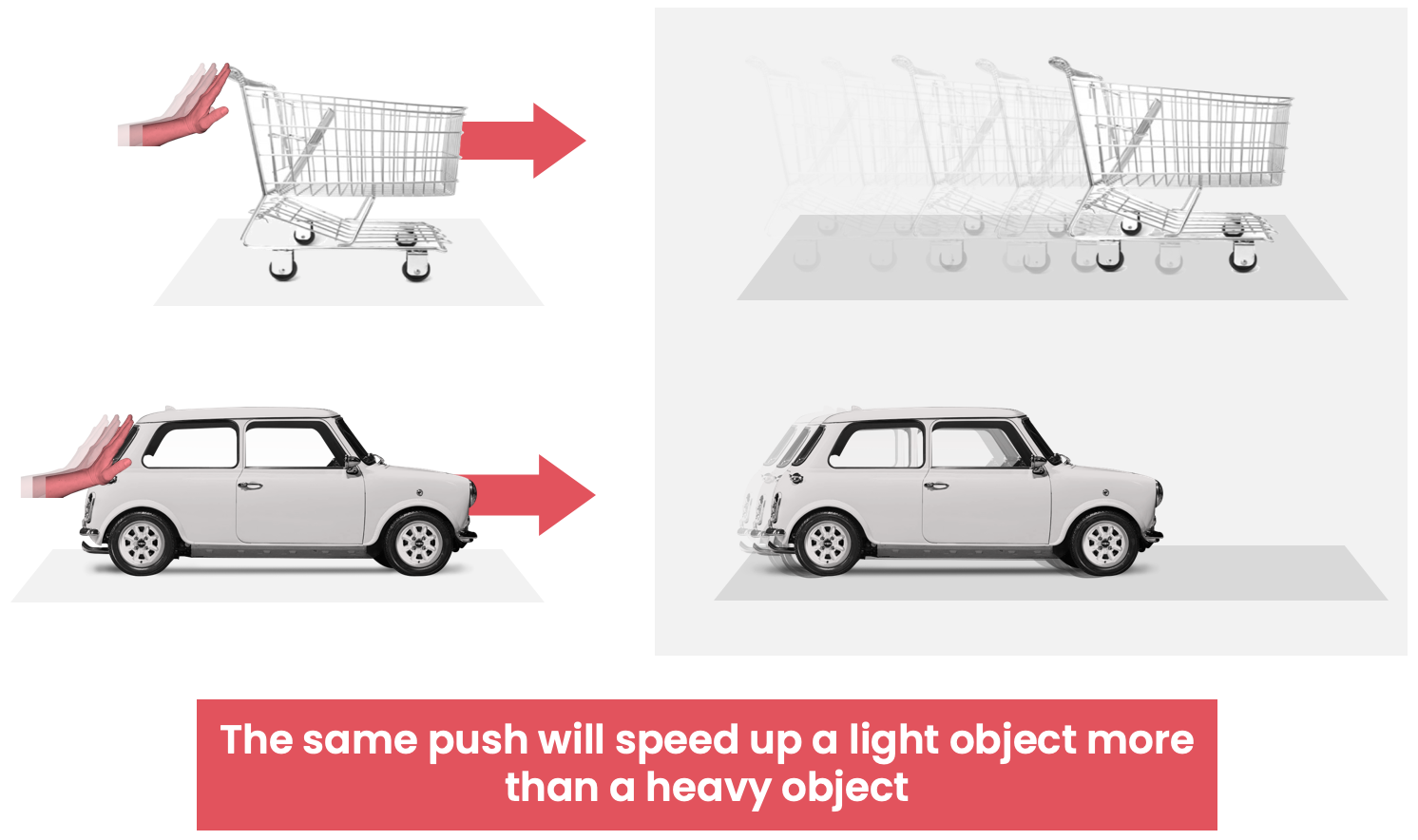Written more scientifically, this is an improvement to the unbalanced force law:

When an object's net force ≠ 0, its speed increases (in the direction of the net force). For an object of double the mass, the speed increase is halved.

This is quite a mouthful! In a few years time, we will study this law much more in-depth (the proper name if this law is Newton's 2nd law of motion).

We have used another word which we don't know yet, mass. We'll learn what mass is on the next page!

Quiz

### Congratulations!

9 of 9 questions completed

+ ⭐️ collected.

• You can change your display name at any time.
• at least 1 letter
• at least 1 number
• at least 8 characters